Next: The Tuple Relational Up: The Relational Algebra Previous: Formal Definition of

1. Additional operations are defined in terms of the fundamental operations. They do not add power to the algebra, but are useful to simplify common queries.

2. The Set Intersection Operation

Set intersection is denoted by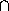, and returns a relation that contains tuples that are in both of its argument relations.

It does not add any power as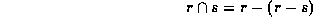To find all customers having both a loan and an account at the SFU branch, we write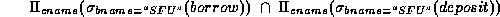3. The Natural Join Operation

Often we want to simplify queries on a cartesian product.

For example, to find all customers having a loan at the bank and the cities in which they live, we need borrow and customer relations: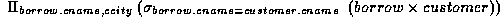Our selection predicate obtains only those tuples pertaining to only one cname.

This type of operation is very common, so we have the natural join, denoted by a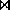sign. Natural join combines a cartesian product and a selection into one operation. It performs a selection forcing equality on those attributes that appear in both relation schemes. Duplicates are removed as in all relation operations.

To illustrate, we can rewrite the previous query as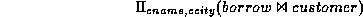The resulting relation is shown in Figure 3.7.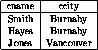Figure 3.7:   Joining borrow and customer relations.

We can now make a more formal definition of natural join.

• Consider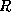and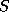to be sets of attributes.
• We denote attributes appearing in both relations by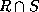.

• We denote attributes in either or both relations by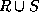.

• Consider two relations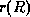and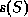.

• The natural join ofand, denoted by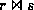is a relation on scheme.
• It is a projection ontoof a selection on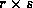where the predicate requires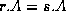for each attribute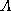in.

Formally,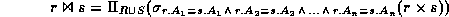where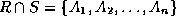.

To find the assets and names of all branches which have depositors living in Stamford, we need customer, deposit and branch relations: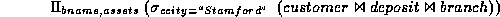Note thatis associative.

To find all customers who have both an account and a loan at the SFU branch: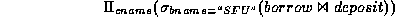This is equivalent to the set intersection version we wrote earlier. We see now that there can be several ways to write a query in the relational algebra.

If two relationsandhave no attributes in common, then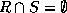, and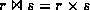.

4. The Division Operation

Division, denoted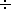, is suited to queries that include the phrase ``for all''.

Suppose we want to find all the customers who have an account at all branches located in Brooklyn.

Strategy: think of it as three steps.

We can obtain the names of all branches located in Brooklyn by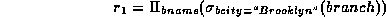Figure 3.19 in the textbook shows the result.

We can also find all cname, bname pairs for which the customer has an account by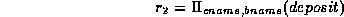Figure 3.20 in the textbook shows the result.

Now we need to find all customers who appear in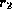with every branch name in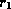.

The divide operation provides exactly those customers: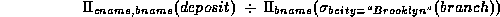which is simply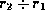.

Formally,

• Letandbe relations.
• Let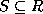.

• The relation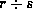is a relation on scheme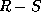.

• A tupleis inif for every tuple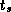inthere is a tuple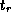insatisfying both of the following: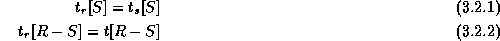• These conditions say that theportion of a tupleis inif and only if there are tuples with theportion and theportion infor every value of theportion in relation.

We will look at this explanation in class more closely.

The division operation can be defined in terms of the fundamental operations.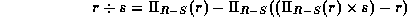Read the text for a more detailed explanation.

5. The Assignment Operation

Sometimes it is useful to be able to write a relational algebra expression in parts using a temporary relation variable (as we did withandin the division example).

The assignment operation, denoted, works like assignment in a programming language.

We could rewrite our division definition as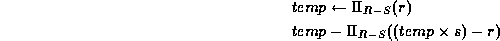No extra relation is added to the database, but the relation variable created can be used in subsequent expressions. Assignment to a permanent relation would constitute a modification to the database.Next: The Tuple Relational Up: The Relational Algebra Previous: Formal Definition of

Page created and maintained by Osmar R. Zaï ane
Last Update: Wed Sep 20 15:45:57 PDT 1995